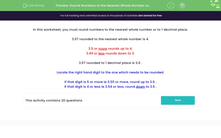# Round Numbers to One Decimal Place

In this worksheet, students will revise and practise rounding numbers to one decimal place.Key stage:  KS 3

Curriculum topic:   Number

Curriculum subtopic:   Round/Approximate Numbers Appropriately

Popular topics:   Rounding worksheets

Difficulty level:#### Worksheet Overview

In this activity, we will round numbers to 1 decimal place.

Example
What is 3.57 rounded to 1 decimal place?

Locate the digit on the right side of the one which needs to be rounded - that means we look at the digit in the hundredths column.

If the digit in the hundredths column is 5 or more, we round the number in the tenths column up.

If the digit in the hundredths column is 4 or less, we round the number in the tenths column down.

In 3.57, the digit in the hundredths column is 7, so we need to round the number in the tenths column up.

3.57 rounded to 1 decimal place is 3.6

If the number we wanted to round had been 3.54, the digit in the hundredths column would have been 4 and the number would have rounded down to 3.5.

Let's have a go at some questions now.### What is EdPlace?

We're your National Curriculum aligned online education content provider helping each child succeed in English, maths and science from year 1 to GCSE. With an EdPlace account you’ll be able to track and measure progress, helping each child achieve their best. We build confidence and attainment by personalising each child’s learning at a level that suits them.

Get started